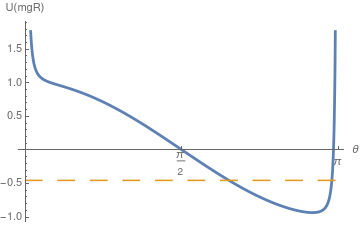# Preface

This section studies some first order nonlinear ordinary differential equations describing the time evolution (or “motion”) of those hamiltonian systems provided with a first integral linking implicitly both variables to a motion constant. An application has been performed on the Lotka--Volterra predator-prey system, turning to a strongly nonlinear differential equation in the phase variables.

Introduction to Linear Algebra with Mathematica

# The Spherical Pendulum

Consider a pendulum bob of mass m hanging from the ceiling by a string of length ℓ and free to move in two dimensions like the Foucault pendulum. This is what is called the spherical pendulum. The free variables are θ and φ of spherical coordinates and the energies are given by
$\begin{split} \Pi &= -mg\ell\,\cos\theta , \\ {\mbox K} &= \frac{1}{2}\,m\ell^2 \left( \dot{\theta}^2 + \sin^2 \theta \,\dot{\phi}^2 \right) . \end{split}$
Then its Lagrangian and Hamiltonian are
$\begin{split} L &= {\mbox K} - \Pi = mg\ell\,\cos\theta + \frac{1}{2}\,m\ell^2 \left( \dot{\theta}^2 + \sin^2 \theta \,\dot{\phi}^2 \right) , \\ H &= {\mbox K} + \Pi = -mg\ell\,\cos\theta + \frac{1}{2}\,m\ell^2 \left( \dot{\theta}^2 + \sin^2 \theta \,\dot{\phi}^2 \right) . \end{split}$
We may calculate the momenta and write the Hamiltonian as a function of them
$\begin{split} p_{\phi} &= \frac{\partial H}{\partial \dot{\phi}} = \frac{\partial {\mbox K}}{\partial \dot{\phi}} = m\ell^2 \sin\theta \,\dot{\phi} , \\ p_{\theta} &= \frac{\partial H}{\partial \dot{\theta}} = \frac{\partial {\mbox K}}{\partial \dot{\theta}} = m\ell^2 \dot{\theta} . \end{split}$
Then the equations of motion become
$\begin{split} \dot{p}_{\phi} &= -\frac{\partial H}{\partial \phi} = 0, \\ &\mbox{ showing that angular momentum about the z-axis is conserved} \\ \dot{p}_{\theta} &= -\frac{\partial H}{\partial \theta} = \frac{p_{\phi}^2 \cos\theta}{m\ell^2 \sin^3 \theta} - mg\ell\,\sin\theta . \end{split}$
Note that the Lagrangian is independent of the angular coordinate φ. It follows that $$\sin^2 \theta \,\dot{\phi}$$ is a constant. As a result, we get the system of differential equations for the spherical pendulum:
$\begin{split} \ddot{\theta} &= \sin\theta\,\cos\theta,\dot{\phi}^2 - \frac{g}{\ell}\,\sin\theta , \\ \frac{\text d}{{\text d}t} \left( \sin^2 \theta \,\dot{\phi} \right) &= 0 \qquad \Longrightarrow \qquad \ddot{\phi} = -2\,\dot{\theta}\dot{\phi}\,\frac{\cos\theta}{\sin\theta} . \end{split}$
Since $$\sin^2 \theta \,\dot{\phi} = p_{\phi}$$ is a constant, we can use the single equation for θ:
$\ddot{\theta} = p_{\phi}^2 \,\frac{\cos\theta}{\sin^3 \theta} - \frac{g}{\ell}\,\sin\theta .$
The total energy is
$E = \frac{1}{2} \left( \dot{\theta}^2 + \frac{p_{\phi}^2}{\sin^2 \theta} \right) + \frac{g}{\ell}\,\cos\theta .$
Let us define an effective potential
$U(\theta ) = \frac{1}{2}\, \frac{p_{\phi}^2}{\sin^2 \theta} + \frac{g}{\ell}\,\cos\theta .$
We define a function for plotting the effective potential, where options may be specified:
effectivePotential[theta0_ /; 0 <= theta0 <= Pi, thetadot0_, phidot0_, opts___Rule] :=
Module[ {pphi}, pphi = (Sin[theta0])^2 phidot0;
Plot[{1/2*(pphi)^2/(Sin[theta])^2 + Cos[theta],
1/2*(thetadot0^2 + (pphi)^2/(Sin[theta0])^2 ) + Cos[theta0] + Cos[theta0]},
{theta, 0 + 10^(-3), Pi - 1/10^3}, Ticks -> {{0, Pi/2, Pi}, Automatic}, AxesLabel -> {"$Theta]", "U(mgR)"}, PlotStyle -> {{Thickness[0.0075]}, {Dashing[{0.05, 0.05}]}}, opts]] Now we plot the effective potential: effectivePotential[2.2, 1.2, -0.1]The potential function. For plotting a spherical pendulum, one may us the following code (there is a bug??). sphericalPendulum[theta0_ /; 0 <= theta0 <= Pi, thetadot0_ /; Abs[thetadot0] < 10, phi0_ /; 0 <= phi0 <= 2*Pi, phidot0_ /; Abs[phidot0] < 10, tmax_ /; 1 <= tmax <= 60, nv_Integer: 999, opts___Rule] := Module[{n = nv, pphi, sol, theta, phi, x, y, z, sphere}, If[n > 1, If[n == 999, n = Round[3 tmax]]; pphi = (Sin[theta0]^2 phidot0 // N); sol = NDSolve[{theta''[t] - Cos[theta[t]]/Sin[theta[t]]^3 + Sin[theta[t]] == 0, phi'[t] == pphi/Sin[theta[t]]^2 , theta = theta0, theta' == thetadot0, phi == phi0}, {theta, phi}, {t, 0, tmax*0.01}, MaxSteps -> 6000]; theta = theta /. First[sol]; phi = phi /. Second[sol]; z[t_] := Cos[theta[t]]; y[t_] := Sin[theta[t]]*Sin[phi[t]]; x[t_] := Sin[theta[t]]*Cos[theta[t]]; sphere = ParametricPlot3D[{{0, Sin[t], Cos[t]}, {Sin[t], 0, Cos[t]}}, {t, 0, 2*Pi}]; ListAnimate[ Table[Show[ sphere, Graphics3D[Line[{{0, 0, -1}, {0, 0, 1}}]], ParametricPlot3D[{x[t], y[t], z[t]}, {t, 0, (tmax/(n - 1))*i + 0.0001}, PlotPoints -> (25 + Round[12*tmax/(n - 1)*i])], Graphics3D[{Thickness[0.0125], Red, Line[{{0, 0, 0}, {x[(tmax/(n - 1))*i], y[(tmax/(n - 1))*i], z[(tmax/(n - 1))*i]}}], PointSize[0.04], Red, Point[{x[(tmax/(n - 1))*i], y[(tmax/(n - 1))*i], z[(tmax/(n - 1))*i]}]}], PlotRange -> {{-1.25, 1.25}, {-1.25, 1.25}}, BoxRatios -> {1, 1, 1}, opts, AxesLabel -> {"x(R)", "y(R)", "z(R)"}], {i, 0, n - 1}]]]] In rectangular coordinates, the position of the bob and its velocities are \[ \begin{cases} x&= \ell\,\sin\theta\,\cos\phi , \\ y&= \ell\,\sin\theta\,\sin\phi , \\ z&= -\ell\,\cos\theta ; \end{cases} \qquad\Longrightarrow \qquad \begin{cases} \dot{x}&= \ell\left( \dot{\theta}\,\cos\theta \,\cos\phi - \dot{\phi}\,\sin\theta\,\sin\phi \right) , \\ \dot{y}&= \ell\left( \dot{\theta}\,\sin\theta \,\cos\phi + \dot{\phi}\,\sin\theta\,\cos\phi \right) , \\ \dot{z} &= \ell \dot{\theta}\,\sin\theta . \end{cases}$
Therefore, the square of the linear velocity is
$v^2 = \dot{x}^2 + \dot{y}^2 + \dot{z}^2 = \ell^2 \left( \dot{\theta}^2 + \dot{\phi}^2 \sin^2 \theta \right) .$
sol = Flatten@ NDSolve[{\[Theta]''[t] == \[Phi]'[t]^2 Cos[\[Theta][t]] -
g/l Sin[\[Theta][t]], \[Phi]''[
t] == (-2 \[Phi]'[t] \[Theta]'[t] Cos[\[Theta][t]])/
Sin[\[Theta][t]], \[Theta] == \[Pi]/2, \[Theta]' ==
0, \[Phi] == \[Pi]/2, \[Phi]' == 1} /. {g -> 9.81, l -> 1}, {\[Theta], \[Phi]}, {t, 0, 10}]
x[t_] := Evaluate[(Sin[\[Theta][t]] Cos[\[Phi][t]]) /. sol]
y[t_] := Evaluate[(Sin[\[Theta][t]] Sin[\[Phi][t]]) /. sol]
z[t_] := Evaluate[Cos[\[Theta][t]] /. sol]
ParametricPlot3D[{x[t], y[t], z[t]}, {t, 0, 10}]

1. Arnol'd, V. I. (1989), Mathematical Methods of Classical Mechanics, Springer-Verlag, ISBN 978-0-387-96890-2
2. Ghorbel, J. and Dabney, J. B., A Spherical Pendulum System to Teach the Concepts in Kinematics, Dynamics, Control, and Simulation, (Aug 19, 2011)